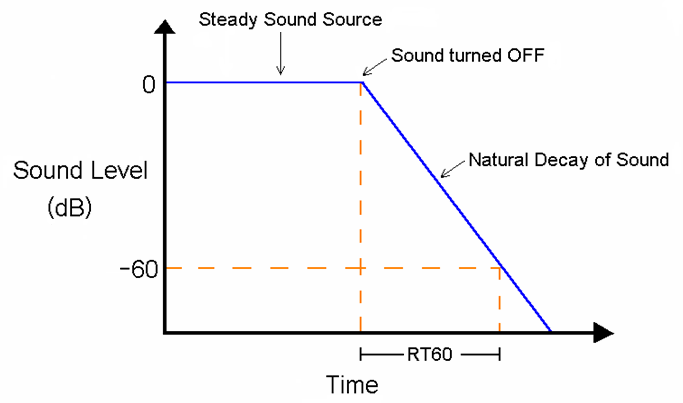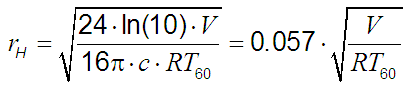Deutsche VersionCalculation of the reverberation time

Sabine's FormulaRT 60 decay

 In 1898, Wallace C. Sabine (Sabin) (1868 - 1919) came up with the reverb time formula, but the article "Collected Papers on Acoustics" appeared in print 1922. Even today his ingenious formula is unchanged in constant use.   Reverberation time RT60 = k · V / A = 0.049 · V / A (V and A in feet)   Reverberation time RT60 = k · V / A = 0.161 · V / A (V and A in meter)   with the factor k  = (24 × ln 10) / c20 = 0.049 (feet) or with k  = (24 × ln 10) / c20 = 0.161 (meter)   RT60 = reverberation time in s (reverb time) V = room volume in m3 A = α · S = equivalent absorption surface or area in m2 α = absorption coefficient or attenuation coefficient The terms "attenuation coefficient" and "absorption coefficient" are used interchangeably S = absorbing surface area in m2 A = α1 · S1 + α2 · S2 + α3 · S3 + .. c20 = speed of sound is 343 m/s or 1126 ft/s at 20°C

 Sound engineers and sound designers ("ear people") mostly use the usual sound field quantity. They therefore state: RT60 is the reverberation time, it takes for the sound pressure level Lp (SPL) to decrease by 60 dB. ● (−)60 dB is equivalent to the thousandth part (10−3) of the initial sound pressure p.   Acousticians and sound protectors ("noise fighters") seem to like more the sound energy quantity. They express this differently: RT60 is the reverberation time, it takes for the sound intensity level LI to decrease by 60 dB. ● (−)60 dB is equivalent to the millionth part (10−6) of the initial sound intensity I.   Sound pressure (sound field quantity) is not sound intensity (sound energy quantity).   I ~ p2   Sometimes you can hear the statement: RT60 is the reverberation time, it takes for the sound level L to drop by 60 dB. Whatever the user wants to tell us so accurately. Level is level or dB is dB.

 Reverberation time (RT) is a measure of the amount of reverberation in a space and equal to the time required for the level of a steady sound to decay by 60 dB after the sound has stopped. The decay rate depends on the amount of sound absorption in a room, the room geometry, and the frequency of the sound. RT is expressed in seconds. This decay is usually measured over the first 10, 20 or 30 dB and then extrapolated to the full 60 dB range. The frequency dependent reverberation time of a room is usually given for the center of a third octave band filter frequency of 500 Hz or 1 kHz or a frequency dependent response curve of the reverberation time of the frequency, but this is not a "frequency response" of the reverberation.   k is a constant that equals 0.161 when the units of measurement are expressed in meters and 0.049 when units are expressed in feet. A = α × S is the total surface absorption A of a room expressed in sabins. It is the sum of all the surface areas in the room multiplied by their respective absorption coefficients. The absorption coefficients α express the absorption factor of materials at given frequencies. Vis the volume of the enclosure. The figures used in this calculator come from the table of absorption coefficients for various materials which can be found in any book that talks about room acoustics.

Enter the measurements of the room
and make sure to specify the units.

 Width Length Height meters feet

125 Hz 250 Hz 500 Hz 1000 Hz 2000 Hz 4000 Hz

Estimated RT 60 of this room is seconds.

Absorption Coefficients α of Building Materials and Finishes

 The reverberation time with the symbol RT60 is the most popular room acoustic parameter that is fundamental. The reverberation time after Wallace C. Sabine (1868 - 1919) is the time interval within which the sound pressure dropped to a value of 10−3, the "thousandth" of its initial value. In practice, the reverberation time is defined as that interval during which the sound pressure level decreases by 60 dB. Acousticians rather consider, however, the sound intensity.The reverberation time is the time interval within which the sound level in a room has faded away by 60 dB. Note: This sound pressure is the thousandth part of the initial sound pressure and the sound intensity is the millionth part of the initial sound intensity. Often this is confusing, because the sound pressure as a sound field quantity is mixed with the sound intensity as a sound energy quantity. Notice: I ~ p2.

Calculating the three room modes − eigenmodes or eigenfrequencies

Standing waves (acoustic resonances)

 Critical distanceV = Room volume in m3 c = Speed of sound is 343 m/s or 1126 ft/s at 20°C RT60 = Reverberation time in s (reverb time) The critical distance rH means D/R = 1